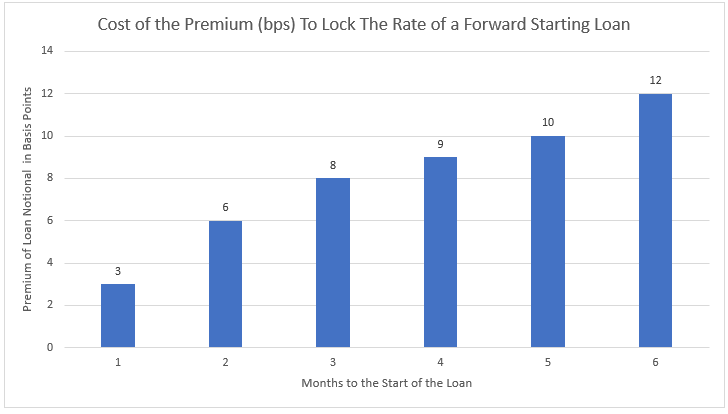In a recent blog [Here], we argued that banks are almost always in an inferior position by not re-quoting the loan rate with market movement until the loan closes. We think that when banks book a fixed-rate loan, the fixed-rate must be finalized at the closing table; otherwise, banks give borrowers a free option that has an economic cost – especially in the current volatile and rising interest rate environment.  However, this article will discuss how banks may properly charge for these forward rate locks and protect their loan margins.

## Using The Forward Curve For Rate Locks

Let’s assume that a bank is pricing a \$1mm, five-year fixed-rate loan using a credit spread of 2.50% over the five-year Treasury rate.  At the time of this writing, the five-year Treasury yield is 1.86%, and the bank would close the loan at a fixed rate equal to 4.36%.  If the bank committed to a 4.36% two months ahead of closing, the bank would take the risk that the five-year Treasury rate could be substantially higher in two months when the loan closes, thereby decreasing the bank’s future net interest margin.The easiest and most straightforward way to price the forward commitment, or “rate lock,” is to look at the forward curve and see where interest rates are expected to be in two months and build that into the pricing.  Currently, the two-month forward rate for five-year Treasuries is six basis points.  The market expects the five-year Treasury in two months to be 1.92%.  The bank can simply price that six basis points premium into the loan to be compensated for that two-month commitment.

## How Volatility Impacts Rate Locks

The problem with the forward premium for pricing a rate lock is that it relies on the market expectation of interest rates and does not factor in the possible dispersion around that expected future rate.  The possibility of rates being substantially higher in two months would cause the bank to lose more than the six basis points currently expected by the forward curve.  For example, in the last 20 days, the high versus low yield for the five-year Treasury yield was 49 basis points.

The cost of this volatility can be priced using an interest rate swaption.  Currently, the cost of a rate lock to cover the volatility (higher rates in two months on five-year Treasury rates) is 2.1% of the loan amount.  Therefore, in our \$1mm, five-year fixed-rate loan example, the bank would need to receive \$21k to cover the economic cost of volatility for two months.  While this rate lock financial cost is actual and measurable to the bank, most borrowers are not willing to pay such a hefty fee to make the bank economically whole.

## Lending Reality

The reality is that banks are almost never correctly compensated in the market for taking the interest rate risk inherent in forward rate locks.  But it is essential to point out that banks that are willing to keep the five-year fixed-rate loan on their balance sheet and take that interest rate risk for the period should not shy away from forward rate locks for two months.  The forward yield premium and the cost of volatility are about the same for a five-year fixed-rate loan and a five-year fixed-rate loan in two months.  In fact, for a bank, the two-month forward on a five-year fixed-rate loan is the same as a 62-month fixed-rate loan.  If the bank is willing to take the on-balance sheet risk of a 62-month fixed-rate loan, it should also be willing to forward rate lock a five-year loan two months out.

## Conclusion

While we are not proponents of fixed-rate loans in this interest rate environment, the risk associated with forward rate locks for a month or two is relatively minimal compared to the cost of carrying a five-year fixed-rate asset.